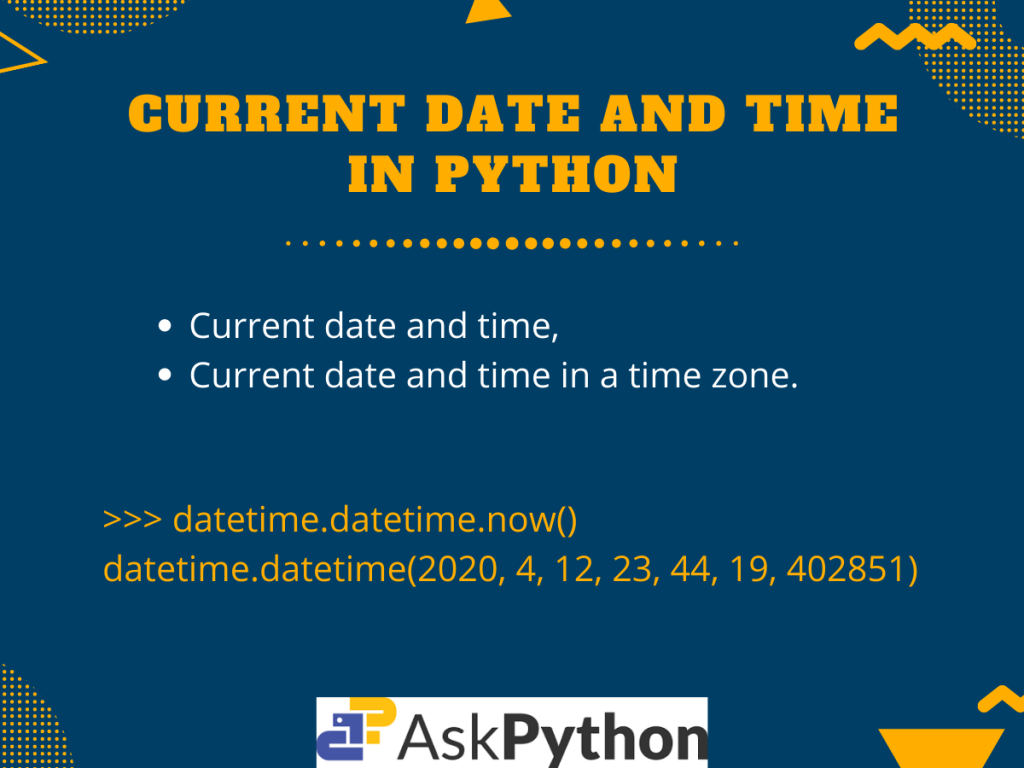# Get Current Date And Time in Python## Introduction

Today in this tutorial, we are going to discuss how we can get the current date and time in Python programming.

Many times a user may need the current system date and time for various operations. This problem can be easily solved by the various built-in methods and modules in Python.

Further, python modules like `pytz` and `pendulum` allow the user to get the current date and time of a specified timezone. This feature is very helpful as it makes the code more reliable.

## Ways to get Current Date And Time in Python

Now let us get right into understanding the different wants to get the current date and time in Python.

### The datetime Module

The `datetime` module supplies classes for manipulating dates and times in Python.

In the example below, we try to fetch the current date using the `today()` method from the date class.

```from datetime import date

today = date.today()
print("Date :", today)
```

Output:

```Date : 2020-04-12
```

Here, `today()` returns the current local date. And simultaneously we print out the date.

Now let us look at another example. This time we are going to print both the local date and current time for the system using the `datetime` module.

```import datetime

#datetime object
dt = datetime.datetime.now()

print("Datetime object =", dt)

# printing in dd/mm/YY H:M:S format using strftime()
dt_string = dt.strftime("Date: %d/%m/%Y  time: %H:%M:%S")
print("Current date and time =", dt_string)
```

Output:

```Datetime object = 2020-04-12 23:16:58.958467
Current date and time = Date: 12/04/2020  time: 23:16:58
```

In the code above,

• The `now()` method from the datetime class returns the current local date and time. Hence, at this point, dt holds the datetime object containing the date and time information
• Nextly, we use the `strftime()` method to convert the dt (datetime object) into a formattable string
• Finally, we print the current date and time in our desired manner

### Using pendulum Module

Pendulum is a timezone library that eases the datetime manipulation. Similar to the `datetime` module, the pendulum module also offers a `now()` method that returns the local date and time.

Pendulum can be easily installed using the PIP command:

```pip install pendulum
```

Example,

```import pendulum
time = pendulum.now()
print(time)
```

Output:

```2020-04-12 23:07:22.940108+05:30
```

As mentioned earlier, the `now()` method returns the current date and time as we can see from the above output.

## Current Date and Time in Python for a Time Zone

When we talk about reliability or cross-platform code usability, Python offers tons of such features. In most of the cases, the user needs date or time for a specific timezone and using some modules like `pytz` or `pendulum` make working in such an environment much easier.

The `pytz` module brings the Olson tz database into Python as well as allows accurate and cross platform timezone calculations.

On the other hand, `pendulum` is a similar library which inherits from the datetime module. It uses a cleaner approach, making it faster than it’s counterpart(`pytz`).

Both the modules can be installed using the following PIP commands,

For `pytz` module:

```pip install pytz
```

For `pendulum` module:

```pip install pendulum
```

### Example

Now let us take an example where we try to get and then print date as well as time for different timezones. This is going to be done using both the `pytz` and `pendulum` module.

```import pytz
from datetime import datetime

utc = pytz.timezone('UTC')
pst = pytz.timezone('America/Los_Angeles')
ist = pytz.timezone('Asia/Calcutta')

print("Using pytz Module:")
print('Current Date and Time in UTC =', datetime.now(utc))
print('Current Date and Time in PST =', datetime.now(pst))
print('Current Date and Time in IST =', datetime.now(ist))

import pendulum

utc1 = pendulum.timezone('UTC')
pst1 = pendulum.timezone('America/Los_Angeles')
ist1 = pendulum.timezone('Asia/Calcutta')

print("Using pendulum Module:")
print('Current Date Time in UTC =', datetime.now(utc1))
print('Current Date Time in PST =', datetime.now(pst1))
print('Current Date Time in IST =', datetime.now(ist1))

```

Output:

Here,

• For both the cases, firstly we use the `timezone()` method defined for both pytz and pendulum modules while passing the required timezone
• Then we pass these timezone objects to the `now()` method from the datetime class. The method returns an accurate date and time for the given timezone. And hence, we directly print it out.

## Conclusion

So in this tutorial we learned about the various ways or techniques to get the current date and time in Python.

For any further questions, feel free to use the comments below.